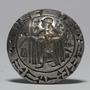1. Forum
2. >
3. Topic: Greek
4. >
5. "Ένας κύκλος δεν έχει μόνο μί…

# "Έναςκύκλοςδενέχειμόνομίαακτίνα."

## Translation:A circle does not have only one radius.

September 7, 2016Plus
•1719

This seems like a very odd thing to say, though it is technically correct. On a diagram of a circle you can have more than one radius. I think the reason why this sounds so strange to me is that a radius on a diagram is quite different from the measure of a radius (which is the the same for all the radii in a circle by definition), which is frequently just called 'the radius' in English. I found it a very confusing sentence to translate into English for this reason.•1221

No, I am still puzzled. If you start from the center of a circle and measure outward you get the radius. You can do this numerous times, but the result will always be the same. These aren't varying radii, but the same radius. A circle really can only have one radius. But hey, I am here to practice Greek, even if the logic is a little wonkyPlus
•1353

In fact the radius pretty much the defines a circle.So we would say that a circle has infinite radii, but all of identical length?

Well, yes, because a circle consists "of all points in a plane that are a given distance from a given point", but those points are not finite.English usage is imprecise between "the line that passes through the centre of the circle and stops at the circumference" and "the length of that line". The former is what is meant in the example sentence here; the latter is what you mean.Plus
•1008

Good point! Merriam Webster includes both the line segment itself and the length of that line segment in the definition of radius. So as to the line segments of any given circle, they are infinite, and as to the length, there is only one.•1304

Why is ...... does not only have one radius marked wrong?

Very strictly speaking, this syntax conveys a different meaning, since the adverb would modify the verb (have) instead of the noun (radius). But they essentially mean the same thing, so it has been added.Can I omit the initial ένας here? Hence

Κύκλος δεν έχει μόνο μία ακτίνα.

Mod
•71

No, that's not possible. Subjects always take an article, definite or indefinite.